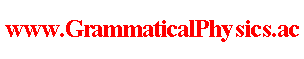since 2006 Help　Sitemap < Forum > < Problems > < Quantum History Theory > < Probability Formula in New Grammar > Let Φ be a normalized functional representing a quantum history.Let the twist remove normalization be used there. Then the function, representing a transition amplitude of the new grammatical quantum mechanics, corresponding to the Green's function of the old quantum mechanics, is given by the following formula. Γ(g, t2; f, t1) = ∫Dχ dar(g; t2, t2 + 1/α)Φ[χ]・{dar(f; t1, t1 + 1/α)Φ[χ]}*.This function corresponds to ＜g, t2| f, t1＞ of the old quantum mechanics. dar(f; a, b) is the stacked Daruma game operator defined as follows. [dar(f; a, b)]pre Φ[χ] = ∫dx f(x)* Φ[ξ(□,x)]where ξ is a function such thatξ(t,x) = χ(t)　(t ＜ a or t ≧ b),ξ(t,x) = x　(a ≦ t < b),ξ(t,x) must be smoothened at t = a and t =b. [dar(f; a, b)] Φ[χ(a,b)] = [dar(f; a, b)]pre Φ[χ]whereχ(a,b)(t) = χ(t)　(t ＜ a),χ(a,b)(t) = χ(t + (b - a))　(t ≧ a). The twist remove normalization for a quantum history written in the form:Φ[χ] = Σj exp[α∫dt φj(χ(t),t)]is defined by the condition:(∂/∂ε)∫dx [exp φj(x,t)]* exp φj(x,t + ε) |ε = 0 = 0.This condition is satisfied with the function:φj(x,t) = φ'j(x,t) + (i/h)t∫dx' [exp φ'j(x',t)]* H exp φ'j(x',t)where exp φ'j is a solution of the old Schrödinger equation and H is the Hamiltonian of it.That is to say,φj(x,t) = φ'j(x,t) + (i/h)t＜j|H|j＞. However to define the twist remove normalization generally is a remaining problem. Twist Remove Normalization --- This article is a rewrite of the article 'Probability Formula in New Grammar' in the following page. Later Edition@Theory of Quantum History Entangled in Time-like Direction@Products of Grammatical Physics@Grammatical Physics@Forum@Vintage(2008-2014) The content of this article was presented by me at JPS 2013 Autumn Meeting. Author Yuichi Uda, Write start at 2015/05/21/19:48JST, Last edit at 2015/05/23/17:50JST